Technical Information Site of Power Supply Design

2019.03.07 DC/DC

# Loss Factors

Examination of Losses

In this article, we examine the relationship between operating conditions and loss increases.

Loss Factors

We have explained that losses occur at various places in a power supply circuit; but the losses at specific places making up the total losses of the circuit become higher depending on the operating conditions. Hence it is necessary to understand the factors that increase losses depending on the operating conditions. Below we have summarized loss factors in relation to various conditions. Calculation formulae for losses are also presented, and should help to further clarify the relation between losses and operating conditions.

Loss Factors that Increase with Increasing Load Current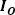・Conduction losses due to the ON-resistance of the high-side MOSFET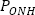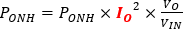・Conduction losses due to the ON-resistance of the low-side MOSFET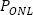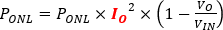・Conduction losses due to the inductor (coil) DCR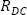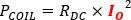Loss Factors that Increase with Higher Frequencies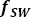・Gate charge losses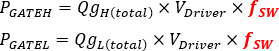Loss Factors that are Affected by Both the Load Currentand the Frequency・Switching losses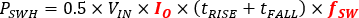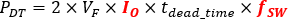These are related to modification of the power supply circuit specifications and changes in the operating conditions. If these relationships are understood, it will be possible to understand what points require attention when re-examining the circuit.

#### Key Points:

・Losses at specific places that make up the overall circuit losses are increased depending on the operating conditions.

・By understanding the loss factors through the equations for the losses, it is possible to understand what points require attention when changing the specifications or the operating conditions.